﻿ 2013~2019年浙江省城乡居民个人卫生支出研究

2013~2019年浙江省城乡居民个人卫生支出研究Study on Personal Health Expenditure of Urban and Rural Residents in Zhejiang Province from 2013 to 2019

Abstract: By studying the GM(1,1) model of personal health expenditure of urban and rural residents in Zhejiang Province, this paper hopes to study the change trend of medical economic burden, provide reference academic value for the formulation of health sector policies in the future, and put forward corresponding countermeasures according to the problems existing in medical economic burden. It is expected that the equity of its health financing structure will be further optimized.

1. 引言

1978年以来，我国医疗卫生改革经历了以“放权让利”为主要方向的探索阶段、以“三项改革”为代表的深化阶段和非典以来的转折调整阶段 。目前“看病难，看病贵”是我国现阶段要解决的迫切问题。为了解决这一问题，科研工作者从各个方面进行了研究工作 。主要研究方向有宏观方面对卫生总费用的研究，还有就某个病症，对患某种特定疾病的患者的经济负担情况做了相关分析 。衡量一个国家的居民是否看病贵，一个重要指标是个人卫生支出，意思是城乡居民在接受各类医疗卫生服务时的直接现金支付，与之相对应的还有政府卫生支出和社会卫生支出，政府卫生支出指各级政府对医疗卫生事业的资金投入，社会卫生支出指政府外的社会各界对卫生事业的资金投入，包括社会医疗保障支出和社会捐赠援助等。本文通过对浙江省的城乡居民个人卫生费用支出进行GM(1,1)模型研究，希望以此研究医疗经济负担变化趋势，为我国未来的卫生部门政策的制定提供参考学术价值，并根据医疗经济负担中存在的问题，提出相应对策，以期其卫生筹资结构公平性将进一步得到优化。

2. 资料来源与方法

2.1. 资料来源

2.2. 研究方法

2.3. 统计学方法

3. 结果

3.1. 基于GM(1,1)模型的个人卫生支出占卫生总费用比例预测Table 1. Personal health expenditure accounts for the proportion of total health expenses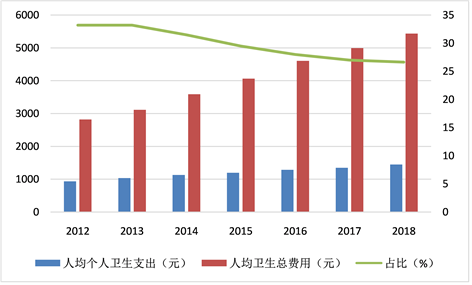Figure 1. The accounting map of average health expenses of urban and rural residents in Zhejiang Province from 2012 to 2018

3.2. 级比检验，建模可行性分析

3.3. 灰色GM(1,1)模型的建立

${Z}^{\left(1\right)}=\left({Z}^{\left(1\right)}\left(2\right),\cdots ,{Z}^{\left(1\right)}\left(7\right)\right)=\left(1452.75,2534.885,3699.035,4942.355,6260.825,7658.705\right)$

$Z=\left(\begin{array}{c}\frac{1}{2}\left[{X}^{\left(1\right)}\left(1\right)+{X}^{\left(1\right)}\left(2\right)\right]\\ \frac{1}{2}\left[{X}^{\left(1\right)}\left(2\right)+{X}^{\left(1\right)}\left(3\right)\right]\\ \frac{1}{2}\left[{X}^{\left(1\right)}\left(3\right)+{X}^{\left(1\right)}\left(4\right)\right]\\ \frac{1}{2}\left[{X}^{\left(1\right)}\left(4\right)+{X}^{\left(1\right)}\left(5\right)\right]\\ \frac{1}{2}\left[{X}^{\left(1\right)}\left(5\right)+{X}^{\left(1\right)}\left(6\right)\right]\\ \frac{1}{2}\left[{X}^{\left(1\right)}\left(6\right)+{X}^{\left(1\right)}\left(7\right)\right]\end{array}\right)=\left(\begin{array}{c}1452.75\\ 2534.885\\ 3699.035\\ 4942.355\\ 6260.825\\ 7658.705\end{array}\right)$

$Y=\left(\begin{array}{c}{X}^{\left(0\right)}\left(2\right)\\ {X}^{\left(0\right)}\left(3\right)\\ {X}^{\left(0\right)}\left(4\right)\\ {X}^{\left(0\right)}\left(5\right)\\ {X}^{\left(0\right)}\left(6\right)\\ {X}^{\left(0\right)}\left(7\right)\end{array}\right)=\left(\begin{array}{c}1034.00\\ 1130.27\\ 1198.03\\ 1288.61\\ 1348.33\\ 1447.43\end{array}\right)$

$B=\left(\begin{array}{c}-{Z}^{\left(1\right)}\left(2\right)\\ -{Z}^{\left(1\right)}\left(3\right)\\ -{Z}^{\left(1\right)}\left(4\right)\\ -{Z}^{\left(1\right)}\left(5\right)\\ -{Z}^{\left(1\right)}\left(6\right)\\ -{Z}^{\left(1\right)}\left(7\right)\end{array}\right)=\left(\begin{array}{cc}-1452.75& 1\\ -2534.885& 1\\ -3699.035& 1\\ -4942.355& 1\\ -6260.825& 1\\ -7658.705& 1\end{array}\right)$

3.4. 模型的拟合检验

GM(1,1)模型需要精度合适才能用以对未来的预测。通常，为了检验预测模型是不是可靠，常用后验差比值法。Table 2. Model accuracy level evaluation standard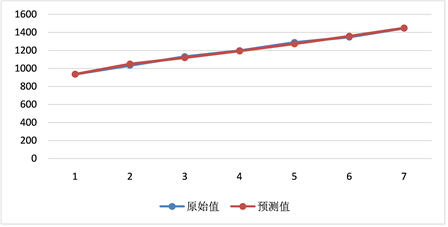Figure 2. Fitting effect map of average health expenses capita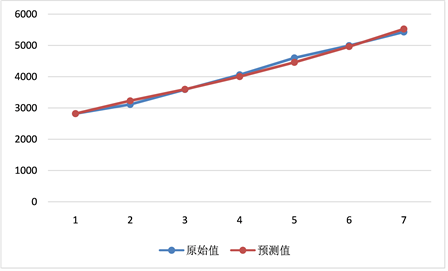Figure 3. Effectiveness map of total capital health expenses

3.5. 对2019年~2023年浙江省人均个人卫生支出进行预测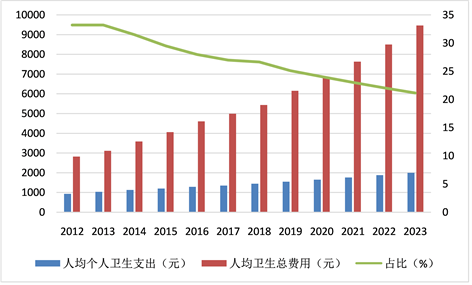Figure 4. Per capita personal health expenditure and accounting map of Zhejiang Province, 2012~2023Table 3. People’s personal health expenditure and its total cost proportion of health expenses in 2019~2023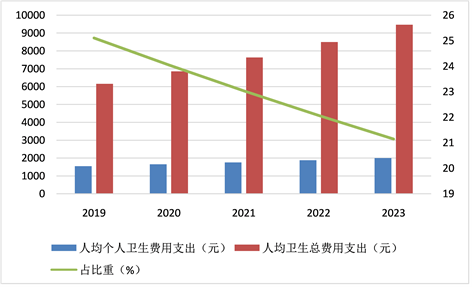Figure 5. Per capita personal health expenditure and accounting map of Zhejiang Province 2019~2023

4. 讨论与结论

1) 结合成本实施医疗，缓解医院趋利性引起的弊端。

2) 提高针对城镇低收入人群和农村高医疗支出人群的医疗补助。

3) 加强对慢性病病用药的管理和控制。随着我国人口老年化的发展与加重，慢性非传染性疾病患病率快速增加，慢病医药费用增长迅速。控制慢性病医药费用，有利于减轻我国人民的医疗经济负担。

4) 鉴于农村医疗经济负担仍然有加重趋势，我国减轻医疗负担仍要以农村为重。有学者就新型农村合作医疗实施后卫生服务可及性和医疗负担的公平性做了研究。结果发现，低收入人群家庭负担依然沉重，对于低收入组这一问题更为突出。因此在以后的工作中，我们要加大政府补贴和投入，建设和完善医疗保障体系，切实减轻农村居民医疗负担。

 李玲, 江宇, 陈秋霖. 改革开放背景下的我国医改30年[J]. 中国卫生经济, 2008, 27(2): 5-9.

 张毓辉, 郭峰, 万泉, 等. 2010年中国卫生总费用测算结果与分析[J]. 中国卫生经济, 2012, 31(4): 5-11.

 刘克军, 王梅. 我国慢性病直接经济负担研究[J]. 中国卫生经济, 2005, 24(10): 77-80.

Top